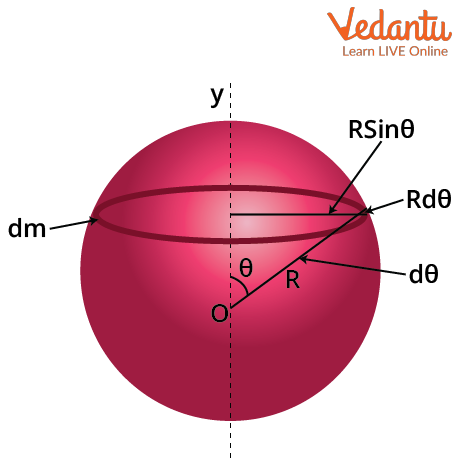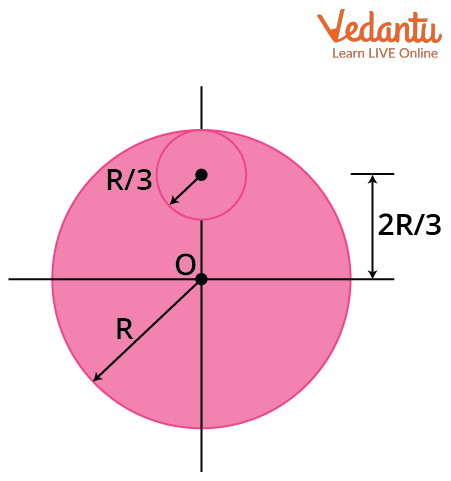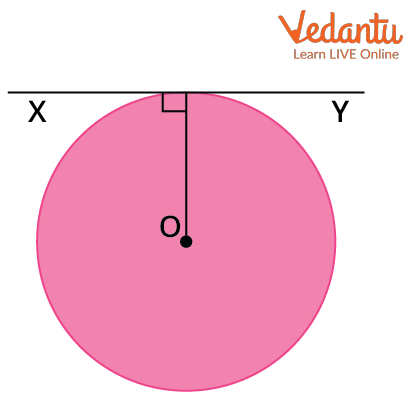Courses
Courses for Kids
Free study material
Offline Centres
More

# Moment of Inertia of a Solid and Hollow Sphere for JEELast updated date: 30th Nov 2023
Total views: 21k
Views today: 0.21k## Moment of Inertia: An Introduction

The moment of inertia is an extremely important topic in rotational mechanics. It is also known as rotational inertia or angular mass. Moment of inertia can be defined as the quantity that expresses the resistance of a body to any angular acceleration or any angular motion in general. It is the sum of the products of the masses of all the particles in the body with the square of the distance of the particle from the axis of the rotation. In more simple terms, it can be defined as the quantity that decides the amount of torque that is needed for a particular angular acceleration in the rotational axis.

The moment of inertia varies with the shape, size, as well as orientation of the rotational axis. It is usually specified for a particular chosen axis of rotation. Changing the axis of rotation also changes the moment of inertia of the object. It basically depends on the distribution of the mass around the axis of rotation.

## What Is a Moment of Inertia?

The moment of inertia is the summation of the product of the masses of all particles to the square of the distance of the particles from the axis of rotation. So, the formula for the moment of inertia is

$I=\sum_{i} m_{i}{r_{i}}{ }^{2}$

This is the moment of the inertia equation.

Here mi is the mass of the particle, ri is the distance of the particle from the rotational axis.

Now the formula above is valid for discrete mass distribution, but if the mass of the system is continuous then the integral form of the moment of inertia is used. The integral form is given as,

$I=\int_{0}^{M} r^{2} \mathrm{~d} m$

The SI unit of the moment of inertia is, kgm2. The dimensional formula of the Moment of Inertia is M1L2T0. Moment of Inertia is also known as angular mass. This is because the moment of inertia serves the same purpose as the mass in angular motion.

## Moment of Inertia of Hollow SphereA Hollow Sphere

Consider M and R to be the mass and the radius of the hollow sphere. O is the centre of the sphere and OY is the axis along which the moment of inertia is to be calculated. Since the sphere is hollow, the mass is spread on the surface.

Let’s consider a thin ring of mass dm. From the diagram, it can be seen that the radius of the ring will be $R\sin{\theta}$. The width of the ring will be $Rd\theta$. The circumference of the ring taken will be $2\pi R\sin{\theta}$. We consider the sphere to be uniform and so the surface mass density will be constant in this case. This means,

$\dfrac{M}{A}=\dfrac{\mathrm{d} m}{\mathrm{~d} a}$

A is the surface area of the sphere which is 4πR2, and we can write da to be equal to the area of the thin disk we considered, and it will be $2 \pi \times R \sin \theta \times R d \theta$. We can write this equation as

\begin{align} &\mathrm{d} m=\dfrac{M \times(2 \pi \times R \sin \theta \times R d \theta)}{4 \pi R^{2}} \\ &\mathrm{~d} m=\dfrac{M}{2} \sin \theta d \theta \end{align}

Now, we can write the equation for the moment of inertia as

\begin{align} &I=\int r^{2} \mathrm{~d} m \\ &I=\dfrac{M}{2} \int_{0}^{\pi}(R \sin \theta)^{2} \sin \theta d \theta ; ~~~\because \theta \text { goes from } 0 \rightarrow \pi \\ &I=\dfrac{M R^{2}}{2} \int_{0}^{\pi} \sin ^{2} \theta \sin \theta d \theta \\ &I=\dfrac{M R^{2}}{2} \int_{0}^{\pi}\left(1-\cos ^{2} \theta\right) \sin \theta d \theta \end{align}

This integral can be solved by taking,

\begin{align} &a=\cos \theta \mathrm{d} \theta \\ &d a=-\sin \theta d \theta \end{align}

When  $\theta=0$, a=1 and similarly when $\theta=\pi$, a=-1. So the limits of the integration will change from 0 to -1.

The integral can now be solved,

\begin{align} &I=\dfrac{M R^{2}}{2} \times \int_{1}^{-1}\left(1-a^{2}\right)(-\mathrm{d} a)\\ &I=\dfrac{M R^{2}}{2} \times \int_{1}^{-1}\left(a^{2}-1\right) \mathrm{d} a\\ &I=\dfrac{M R^{2}}{2} \times\left(\dfrac{a^{3}}{3}-a\right)_{1}^{-1}\\ &I=\dfrac{M R^{2}}{2} \times\left(\dfrac{-1}{3}+1-\frac{1}{3}+1\right)\\ &I=\dfrac{M R^{2}}{2} \times\left(-\dfrac{2}{3}+2\right)\\ &I=\dfrac{M R^{2}}{2} \times\left(\dfrac{-2+6}{3}\right)\\ &I=\dfrac{M R^{2}}{2} \times\left(\dfrac{4}{3}\right)\\ &I=\dfrac{2 M R^{2}}{3} \end{align}

This is the moment of inertia of a hollow sphere.

## Moment of Inertia of a Solid Sphere

We’ll be calculating the moment of inertia of a solid sphere about its diameter. Let’s consider a uniform solid sphere of mass M and radius R. We can calculate the moment of inertia of this solid sphere using the moment of inertia of a hollow sphere. The moment of inertia of a hollow sphere is given as

$I=\dfrac{2}{3} M R^{2}$

Now, if we consider infinite concentric hollow spheres of different radii, they’ll eventually form a solid sphere. So the infinitesimal moment of inertia of a hollow sphere can be written as,

$d I=\dfrac{2}{3} r^{2} d m$...(1)

Now if we integrate this we’ll get the moment of inertia of a solid sphere. Since we have taken a uniform solid sphere, the volume density will be constant. So we can write

$\dfrac{M}{V}=\dfrac{d m}{d V}$

Then substituting the volume of both the solid sphere and the thin spherical shell, we get

\begin{align} &\dfrac{M}{\dfrac{4}{3} \pi R^{3}}=\dfrac{d m}{4 \pi r^{2} \cdot d r} \\ &d m=\dfrac{3 M}{R^{2}} r^{2} d r \end{align}

Substituting dm in (1) gives

\begin{align} &\int d I=\dfrac{2}{3} \int r^{2} \dfrac{3 M}{R^{3}} r^{2} \mathrm{~d} r \\ &\int d I=\dfrac{2 M}{R^{3}} \int r^{4} \mathrm{~d} r \end{align}

Integrating the above equation from 0 to R will give the moment of inertia of a solid sphere.

\begin{align} &I=\dfrac{2 M}{R^{3}} \int_{0}^{R} r^{4} \mathrm{~d} r \\ &I=\dfrac{2 M}{R^{3}}\left(\dfrac{r^{5}}{5}\right)_{0}^{R} \\ &I=\dfrac{2 M}{R^{3}}\left(\dfrac{R^{5}}{5}-0\right) \\ &I=\dfrac{2 MR^{2}}{5} \end{align}

This is the moment of inertia of a solid sphere about its diameter. The moment of inertia is usually written as moi, so the above equation can be called as the moi of the solid sphere formula.

## Numerical Examples on Moment of Inertia

Example 1: From a uniform circular disc of radius R and mass 9M, a small disc of radius R/3 is removed as shown in the figure below. Find the moment of inertia of the remaining disc about an axis perpendicular to the plane of the disc and passing through the centre of the disc.Moment of Inertia

Solution: Mass of the disc is given to be 9M. Since this is a uniform disc, the mass density will be constant. Using this we can find the mass of the removed disc. Let’s consider the mass of the removed disc to be m. We can write

\begin{align} &\dfrac{9 M}{\pi R^{2}}=\dfrac{m}{\pi\left(\dfrac{R}{3}\right)^{2}} \\ &\dfrac{9 M}{\pi R^{2}}=\dfrac{9 m}{\pi R^{2}} \\ &M=m \end{align}

So the mass of the removed portion is M.

We know that the moment of inertia of the complete disc about an axis that passes through its centre and is perpendicular to its plane is $\dfrac{1}{2} M R^{2}$

For the given complete disc, M=9M and R=R, so the moment of inertia will be,

$I_{a}=\dfrac{9}{2} M R^{2}$

Now the moment of inertia of the removed  disc of mass M and radius $\dfrac{R}{3}$ will be

\begin{align} &I_{b}=\dfrac{1}{2} M\left(\dfrac{R}{3}\right)^{2} \\ &I_{b}=\dfrac{1}{18} M R^{2} \end{align}

Using the parallel axis theorem we can now find the moment of inertia of this removed disc about the centre O. This will be the sum of the moment of inertia of the disc about its own axis and the product of the mass and the square of distance between both axes. The distance between the centre of the disc to be removed and the complete disc is $\dfrac{2R}{3}$. So the moment of inertia of the disc of radius $\dfrac{R}{3}$ about the axis passing through O is,

\begin{align} &I_{c}=\dfrac{1}{18} M R^{2}+M\left(\dfrac{2 R}{3}\right)^{2} \\ &I_{c}=\dfrac{1}{18} M R^{2}+\dfrac{4}{9} M R^{2} \\ &I_{c}=\dfrac{(1+8)}{18} M R^{2} \\ &I_{c}=\dfrac{1}{2} M R^{2} \end{align}

So the moment of inertia of the remaining disc will be the difference between the moment of inertia of the complete disc and the moment of inertia of the disc of radius $\dfrac{R}{3}$ both taken from an axis passing through the centre O and perpendicular to the plane.

\begin{align} &I=I{ }_{a}-I_{c} \\ &I=\dfrac{9}{2} M R^{2}-\dfrac{1}{2} M R^{2} \\ &I=4 R^{2} \end{align}

So the moment of inertia of the remaining disc about an axis perpendicular to the plane of the disc and passing through the centre of the disc is equal to 4MR2.

Example 2: A thin wire of length L and uniform linear mass density ρ is bent into a circular loop with centre at O as shown in the figure. Find the moment of inertia of the loop about an axis XY shown in the figure.Moment of Inertia

Solution: The length of the wire will be equal to the circumference of the circle. So if we consider R to be the radius of the loop then

\begin{align} &2 \pi R=L \\ &R=\dfrac{L}{2 \pi} \end{align}

Also if mass of the wire is m then the linear mass density is given as

\begin{align} &\dfrac{m}{L}=\rho \\ &m=\rho L \end{align}

The moment of inertia of a circular ring about its tangent is given as

$I=\dfrac{3}{2} M R^{2}$

We have the values of M and R for our given circle. So the moment of inertia will be,

\begin{align} &I=\dfrac{3}{2}(\rho L)\left(\dfrac{L}{2 \pi}\right)^{2} \\ &I=\dfrac{3}{2} \rho L \times \dfrac{L^{2}}{4 \pi^{2}} \\ &I=\dfrac{3}{8 \pi^{2}} \rho L^{3} \end{align}

Hence, the moment of inertia of the loop about an axis XY is $\dfrac{3}{8 \pi^{2}} \rho L^{3}$.

## Conclusion

The quantity that expresses a body's resistance to any angular acceleration or any angular motion, in general, is known as the moment of inertia. It is the sum of the masses of all the particles in the body multiplied by the square of the particle's distance from the rotation axis. The formula for the moment of inertia is

$I=\sum_{i} m_{i} r_{i}^{2}$.

The integral form of the moment of inertia formula is

$I=\int_{0}^{M} r^{2} \mathrm{~d} m$

The formula for the moment of inertia of a hollow sphere is

$I=\dfrac{2}{3} M R^{2}$

The formula for the moment of inertia of a solid sphere is

$I=\dfrac{2}{5} M R^{2}$.

## FAQs on Moment of Inertia of a Solid and Hollow Sphere for JEE

1. What is the weightage of the topic moment of inertia in JEE Main?

Moment of inertia comes under the chapter of rotational motion in mechanics. Rotational motion has a weightage of about 3.3% in the JEE Main exam and every year 1 question is asked from this topic. In rotational motion, moment of inertia is extremely important as a variety of questions can be framed from this topic. Not just in JEE Main, rotational motion and moment of inertia are important topics for JEE Advance too.

2. Why does a solid sphere have a smaller moment of inertia than a hollow sphere of the same mass and radius, about an axis passing through their axes of symmetry?

For a hollow sphere, all the mass of the sphere is on the surface of the sphere, whereas for a solid sphere the mass is distributed throughout the volume of the sphere. For a hollow sphere, all particles are R away from the axis and hence contribute the same amount to the moment of inertia. However, some particles in a solid sphere are closer to R than others, therefore their contribution to the moment of inertia is lower.